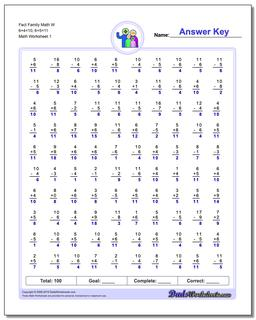# Math Worksheets: Fact Family Math: Fact Family Math: Fact Family Math W 6+4=10, 6+5=11## Fact Family Math W 6+4=10, 6+5=11

PropertyValue
DescriptionFact Family Math W 6+4=10, 6+5=11: These math worksheets have 100 addition and subtraction fact family problems and make for a challenging two minute test.
Resource TypeWorksheet